# AP Statistics : Properties of Single Sample Distributions

## Example Questions

← Previous 1

### Example Question #1 : Properties Of Single Sample Distributions

A researcher wants to determine whether there is a significant linear relationship between time spent meditating and time spent studying. What is the appropriate null hypothesis for this study?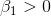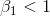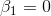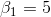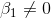Explanation:

This question is about a linear regression between time spent meditating and time spent studying. Therefore, the hypothesis is regarding Beta1, the slope of the line. We are testing a non-directional or bi-directional claim that the relationship is significant. Therefore, the null hypothesis is that the relationship is not significant, meaning the slope of the line is equal to zero.

Explanation:

Explanation:

### Example Question #4 : Properties Of Single Sample Distributions

The president of a country is trying to estimate the average income of his citizens. He randomly samples residents and collects information about their salaries. A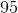percent confidence interval computed from this data for the mean income per citizen is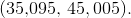Which of the following provides the best interpretation of this confidence interval?

There is apercent probability that the mean of another sample with the same size will fall between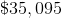and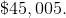percent of the citizens of the country have an income that is betweenandThere is apercent probability that the mean income per citizen in the school is betweenandIf he was to take another sample of the same size and compute apercent confidence interval, we would have apercent chance of getting the intervalThere is apercent probability that all the citizens of the country have an income  betweenandThere is apercent probability that the mean income per citizen in the school is betweenandExplanation:

A confidence interval is a statement about the mean of the population the sample is drawn from so there is apercent probability that apercent confidence interval contains the true mean of the population.

### Example Question #5 : Properties Of Single Sample Distributions

Assume you have taken 100 samples of size 64 each from a population. The population variance is 49.

What is the standard deviation of each (and every) sample mean?

.875

.9

.7

.65

.35

.875

Explanation:

The population standard deviation =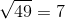The sample mean standard deviation =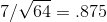### Example Question #6 : Properties Of Single Sample Distributions

Reaction times in a population of people have a standard deviation ofmilliseconds. How large must a sample size be for the standard deviation of the sample mean reaction time to be no larger thanmilliseconds?Explanation:

Use the fact that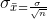.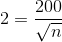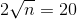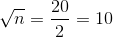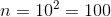Alternately, you can use the fact that the variance of the sample mean varies inversely by the square root of the sample size, so to reduce the variance by a factor of 10, the sample size needs to be 100.

### Example Question #7 : Properties Of Single Sample Distributions

A machine puts an average ofgrams of jelly beans in bags, with a standard deviation of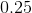grams.bags are randomly chosen, what is the probability that the mean amount per bag in the sampled bags is less than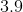grams.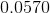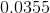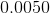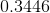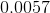Explanation:

A sample size ofbags means that the central limit theorem is applicable and the distribution can be assumed to be normal. The sample mean would be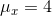and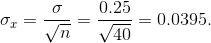Therefore,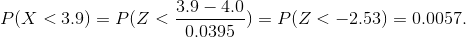### Example Question #8 : Properties Of Single Sample Distributions

Which of the following is a sampling distribution?

The height of a particular college student.

The average height of all college students.

The distribution of average height statistics that could happen from all possible samples of college students.

The average height of a sample of college students.

The distribution of average height statistics that could happen from all possible samples of college students.

Explanation:

The correct answer is the distribution of average height statistics that could happen from all possible samples of college students. Remember that a sampling distribution isn't just a statistic you get form taking a sample, and isn't just a piece of data you get from doing sampling. Instead, a sampling distribution is a distribution of sample statistics you could get from all of the possible samples you might take from a given population.

### Example Question #9 : Properties Of Single Sample Distributions

If a sampling distribution for samples of college students measured for average height has a mean of 70 inches and a standard deviation of 5 inches, we can infer that:

Roughly 68% of college students are between 65 and 75 inches tall.

Any particular random sample of college students will have a mean of 70 inches and a standard deviation of 5 inches.

Roughly 68% of random samples of college students will have a sample mean of between 65 and 75 inches.

College students are getting shorter.

Roughly 68% of random samples of college students will have a sample mean of between 65 and 75 inches.

Explanation:

We can infer that roughly 68% of random samples of college students will have a sample mean of between 65 and 75 inches. Anytime we try to make an inference from a sampling distribution, we have to keep in mind that the sampling distribution is a distribution of samples and not a distribution about the thing we're trying to measure itself (in this case the height of college students). Also, remember that the empirical rules tells us that roughly 68% of the distribution will fall within one standard deviation of the mean.

### Example Question #10 : Properties Of Single Sample Distributions

The standard deviation of a sampling distribution is called:

Standard error

John McEnroe

Sample variance

Sampling deviation